Disclaimer: This is an example of a student written essay.

Any scientific information contained within this essay should not be treated as fact, this content is to be used for educational purposes only and may contain factual inaccuracies or be out of date.

# Theory of Two Magnetically Coupled RLC Circuits

 ✅ Paper Type: Free Essay ✅ Subject: Physics ✅ Wordcount: 3128 words ✅ Published: 9th Mar 2018

CHAPTER 3

In many physical situations coupling can be created between two or more oscillatory systems. For instance, two pendulum clocks which are mounted on the same wall will be coupled by the flexing of the wall as each swing. Similarly, electronic devices frequently contain several tuned circuits that may be deliberately coupled by another circuit element, or even accidentally by stray fields. In all these situations, energy can be transferred when the frequency of one or both of the oscillators will be shifted. (Anon, 2011)

3.1 Theory of two magnetically coupled RLC circuits

Two inductively coupled RLC circuits are shown below (Figure 1). Two resonant frequencies are obtained owing to the fact that there are two circuits. The separation of the two frequencies depends on the value of the mutual inductance M, defined as the ratio of the

voltage in the secondary to the rate of change of primary current with time. It has a reactance at the operating frequency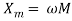. (Arkadi, 2004)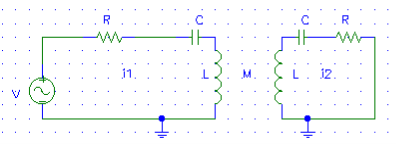Applying Kirchhoff’s voltage law equations for both the primary and secondary loops yield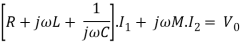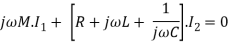(it is assumed here that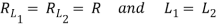)

These equations can be written in the matrix form as follows: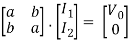where,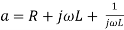and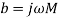Following Cramer’s rule,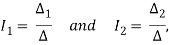where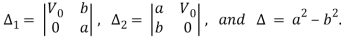Thus, the solution from which the frequency response can be obtained is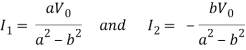Resonance occurs at the two frequencies given by the following equations: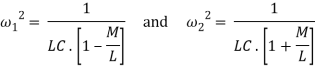The behavior of the circuit can qualitatively be understood on the basis of the reflected impedance (or coupled impedance). An inductively coupled circuit is said to “reflect” impedance in the secondary into the primary circuit. For a further explanation, the coupled circuits shown in Fig 2, is considered.

The positive direction of the currents is chosen into the polarity mark on the generator representing the induced voltages, so that Kirchhoff’s equations are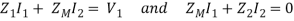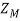is the mutual impedance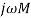,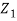includes the source impedance and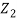the secondary load. These equations may be solved for the equivalent primary impedance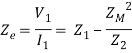The reflected impedance is then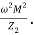A resistance is reflected as a resistance, whereas a capacitance is reflected as an inductance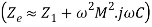, and an inductance reflected as a capacitance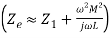.

At resonance condition, the reflected impedance is resistive, and therefore acts to lower the Q-factor of the primary, and thereby reducing the output. This is however counteracted by an increase in coupling, which increases the output. The lower Q-factor gives a wider bandwidth. At lower frequencies than exact resonance, the reflected impedance is said to be inductive, which contributes to the inductance of the primary and consequently resonates at a lower frequency, producing a peak in the output. At higher frequencies than exact resonance, the reflected impedance is said to be capacitive, which cancels part of the inductance and eventually causes the circuit to resonate at a higher frequency, producing the second peak. (Arkadi, 2004)

3.2 Theory of couplings between two resonators

The operation of resonators is very similar to that of the lumped-element resonators (series and RLC resonant circuits). Generally, two eigen frequencies can be obtained in association with the coupling between two coupled resonators, despite whether ther are synchronously or asynchronously tuned. The coupling coefficient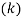, can therefore be extracted from these two frequencies, which can be obtained using eqn () and eqn (). However, these two frequencies can also be easily and directly identified in experiments without doing any calculations.

According to (Hong, 2004), the formula for the computation of the coupling coefficient for synchronously tuned resonators does not yield the appropriate results when used to compute the coupling coefficient of asynchronously tuned resonators. Therefore it is of fundamental importance to present comprehensive treatment and derive a proper formulation to extract the coupling coefficient for asynchronously tuned resonators.

In general, for different structure resonator (Figure ), the coupling coefficient may have different self-resonant frequencies. It may be defined on the basis of a ratio of coupled energy to stored energy, that is,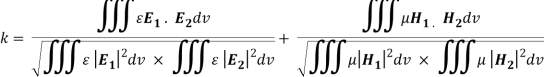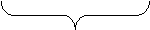Electric coupling magnetic coupling

where all fields are determined at resonance. The volume integrals are over entire regions with permittivity of Æ and permeability of μ. However the direct evaluation of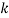from eqn. would require a complete knowledge of the field distributions and would need to perform space integral. This would certainly not be an easy piece of work unless analytical solutions of the fields exist.

However, Hong et al. (2004) found that there exists a relation between the coupling coefficient and resonant frequencies of the resonators which eases our task in computing the coupling coefficient.The coupling is due to both electric and magnetic effects. It is therefore essential to formulate expressions for each type of coupling separately.

3.3 Formulation for coupling coefficients

3.3.1 Electric coupling

For electric coupling alone, an equivalent lumped-element circuit (Figure ) is designed to represent the coupled resonators. The two resonators resonate at frequencies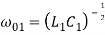and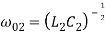. They are coupled to each other through mutual capacitance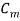. For natural resonance to occur, the condition is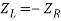(as mentioned previously in 2.2.3). The resonant condition leads to an eigen equation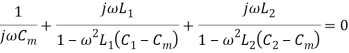After some manipulations eqn () reduces to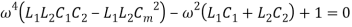This equation has four eigenvalues or solutions. However, out of the four, only the two positive real solutions are of interest to us. This is because they represent the resonant frequencies which are identifiable, namely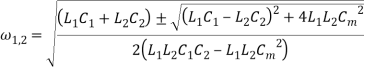A new parameter is defined,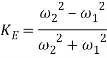where it is assumed that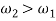.

Substitutingandin eqn (),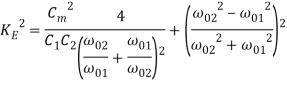Defining the electric coupling coefficient,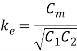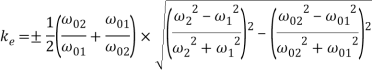according to the ratio of the coupled electric energy to the average stored energy.

3.3.2 Magnetic coupling

A lumped-element circuit model like Figure is used to show the magnetic coupling through mutual inductance,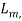of asynchronously tuned resonators.andare the two resonant frequencies of the uncoupled resonators. For natural resonance to occur, the condition is,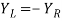.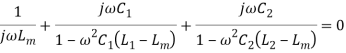After expanding,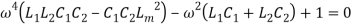Like in 3.3.1, this equation has four solutions, of which only the two positive real ones are of interest to us,We define a parameter,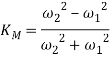Assuming, and recallingand, substitute in eqn ()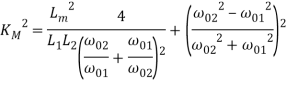Defining the magnetic coupling coefficient as the ratio of the coupled magnetic energy to the average stored energy,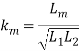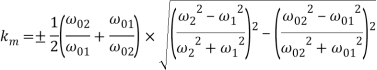3.3.3 Mixed coupling

There is a mixture of both electric and magnetic coupling in the case of the experiments that will be performed in this project. Therefore to derive the coupling coefficient of the two resonators, we may have a circuit model as shown in Fig.Fig.

The electric coupling is represented by an admittance inverter with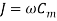while the magnetic coupling is represented by an impedance inverter with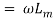.

Based on the circuit model of Fig. , and assuming all internal currents flow outward each node, a definite nodal admittance matrix can be define with a reference at node ‘0’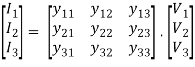with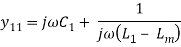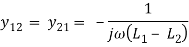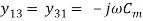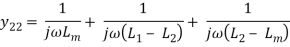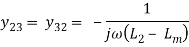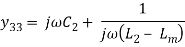For natural resonance, it implies that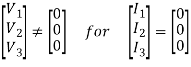This requires that the determinant of admittance matrix to be zero, that is,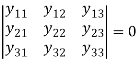After some manipulations, we can arrive at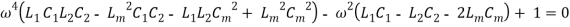This biquadratic equation is the eigen-equation for an asynchronously tuned coupled resonator circuit with the mixed coupling. Letting either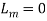or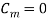in eqn. reduces the equation to either coupling, which is what should be expected. There are four solutions of eqn. However, only the two positive ones are of interest, and they may be expressed as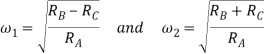with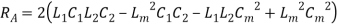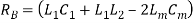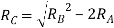Define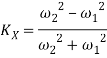For narrow-band applications we can assume that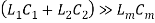and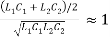the latter actually represents a ration of an arithmetic mean to a geometric mean of two resonant frequencies. Thus we have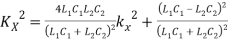in which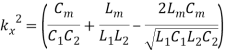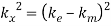Now, it is clear that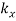is nothing else but the mixed coupling coefficient defined as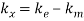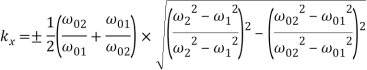The derived formula for extracting the coupling coefficients of any two asynchronously resonators can thus be formulated as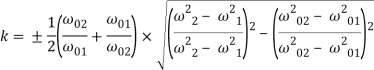This formula can also be used in computing the coupling coefficient of two synchronously tuned resonators, and in that case it reduces to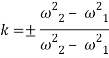We will demonstrate the application of the derived formulation in this project through the construction of two identical coupled spiral coil resonators and identify their respective resonant frequencies as well as determining the mixed coupling between them through the use of capacitors added to them.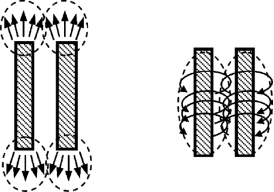View all

## DMCA / Removal Request

If you are the original writer of this essay and no longer wish to have your work published on UKEssays.com then please:

Related Services

Prices from

BHD57

Approximate costs for:

• 1000 words
• 7 day deliveryHumanity University

Dedicated to your worth and value as a human being!

Related Lectures